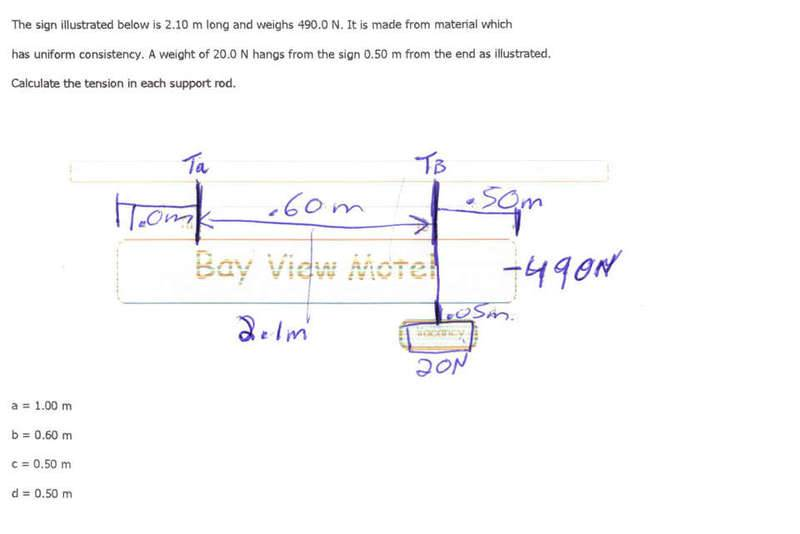# Can someone HELP Finding Tension

## Homework Statement

Heres the question:The picture is not to scale
I am suppose to find the tension of both Ta and Tb

I have posted this question several time on different sites and no one seems to be able to answer it for me...If you can show me the process in doing this question I would REALLY appreciate it.

Thanks

## Answers and Replies

Delphi51
Homework Helper
It should be easy to find the total of the two tensions - the force necessary to hold the whole thing up.

Finding one of them independently should be possible using moments (torques) about some cleverly chosen point. My guess would be to use the point where the right hand rope is attached.

I tried the question and this is what I got:
(490)(.55)=(Ta)(.60)
269.5=(Ta)(.60)
269.5/.60=Ta
Ta=449.167

Tb=490-449.167
Tb=40.83+20
Tb=60.83

So
Ta=449.167
Tb=60.83

Im not sure if im right...what do you think

Delphi51
Homework Helper
Agree!